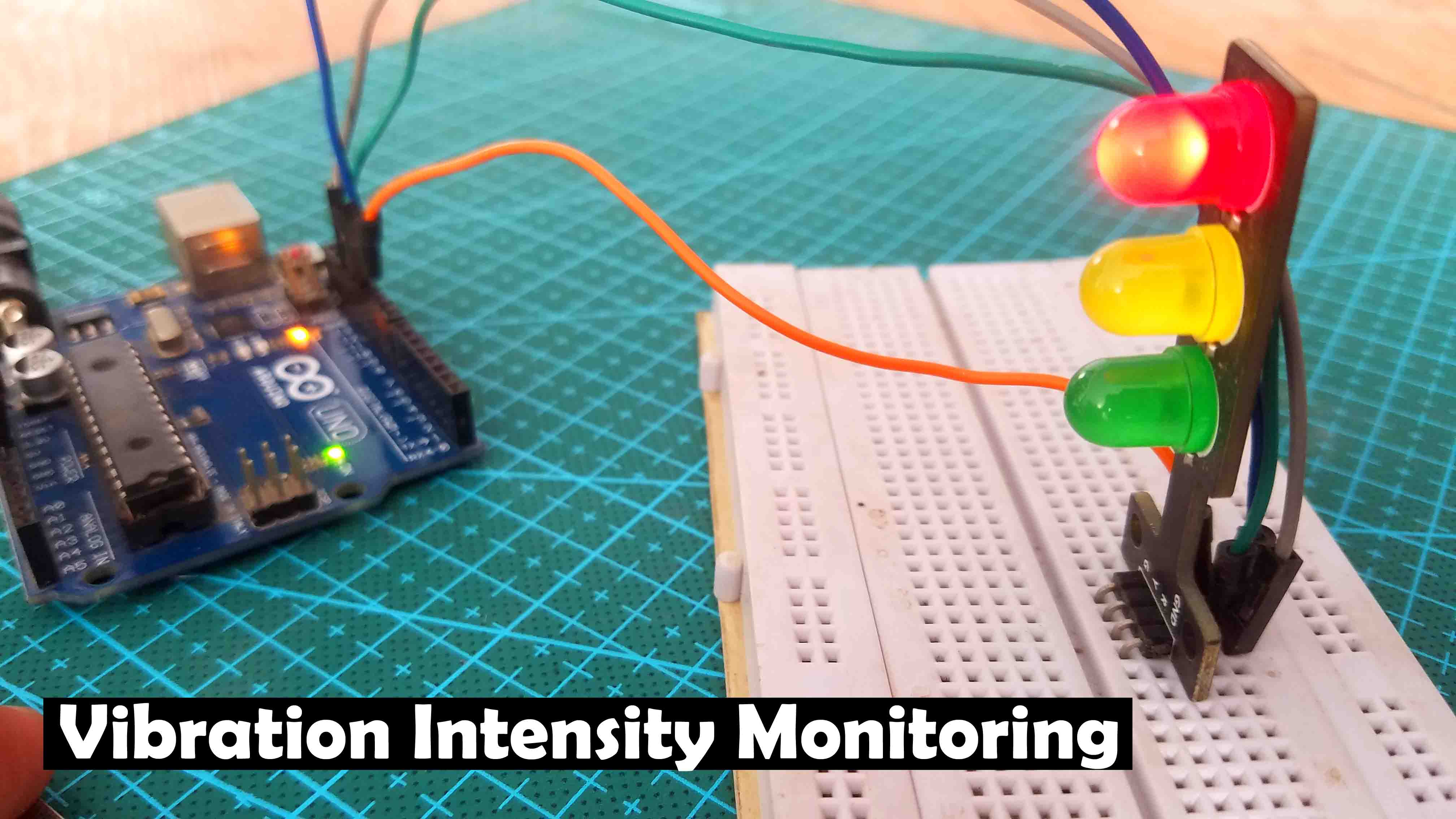# Vibration Sensor with Arduino, Vibration Detector AAC 51-000923

(Last Updated On: September 9, 2021)

## Vibration Sensor with Arduino: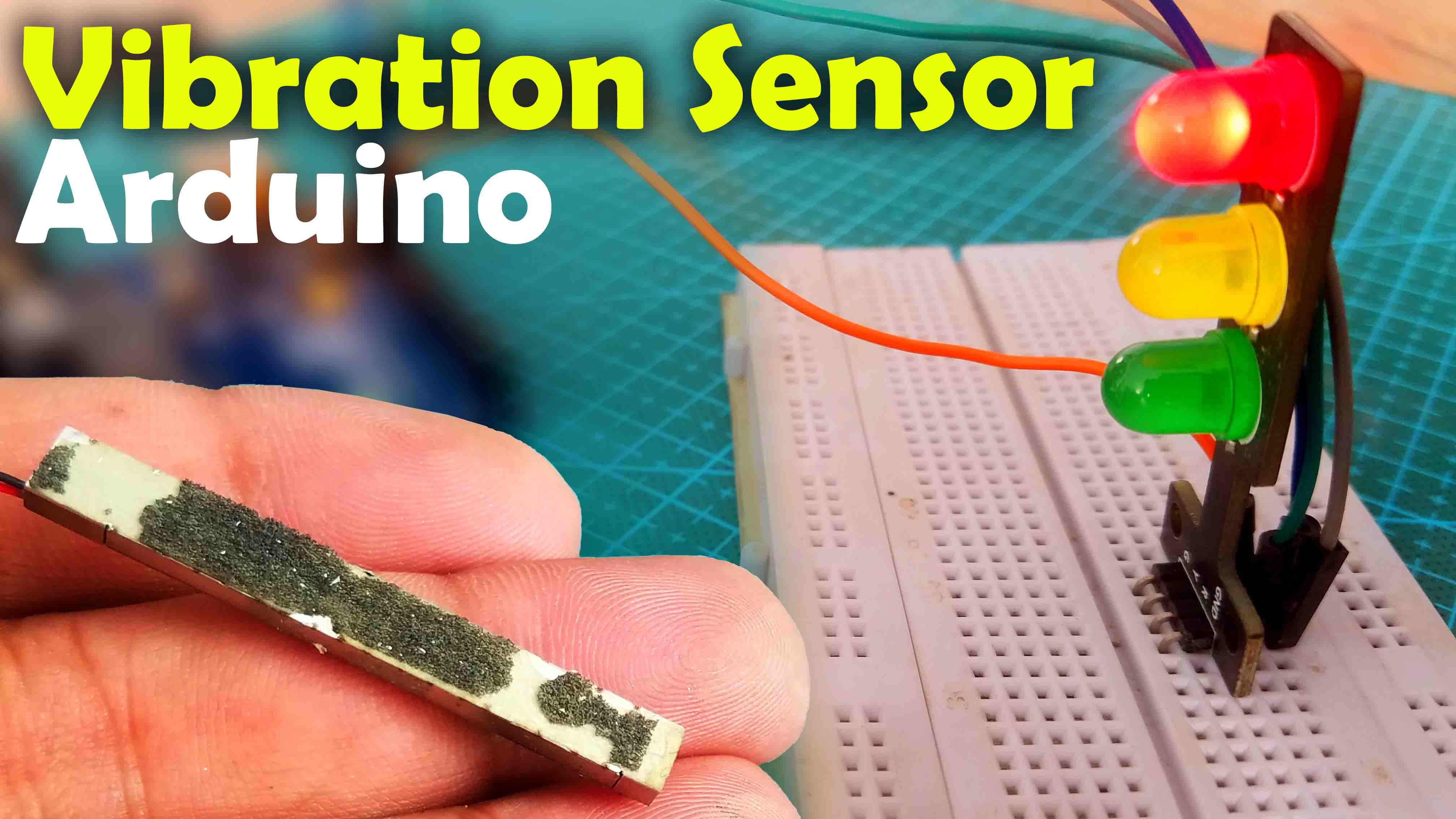Vibration Sensor with Arduino- in this tutorial, we are going to be looking at this new type of the Vibration Sensor which is quite different from the one I used in my previous projects for detecting the vibration.This is the SW-420 Vibration Sensor and I have used this Vibration sensor with the Arduino and also with the Nodemcu ESP8266 WiFi Module for monitoring the Vibration wirelessly from anywhere around the world using the Blynk application.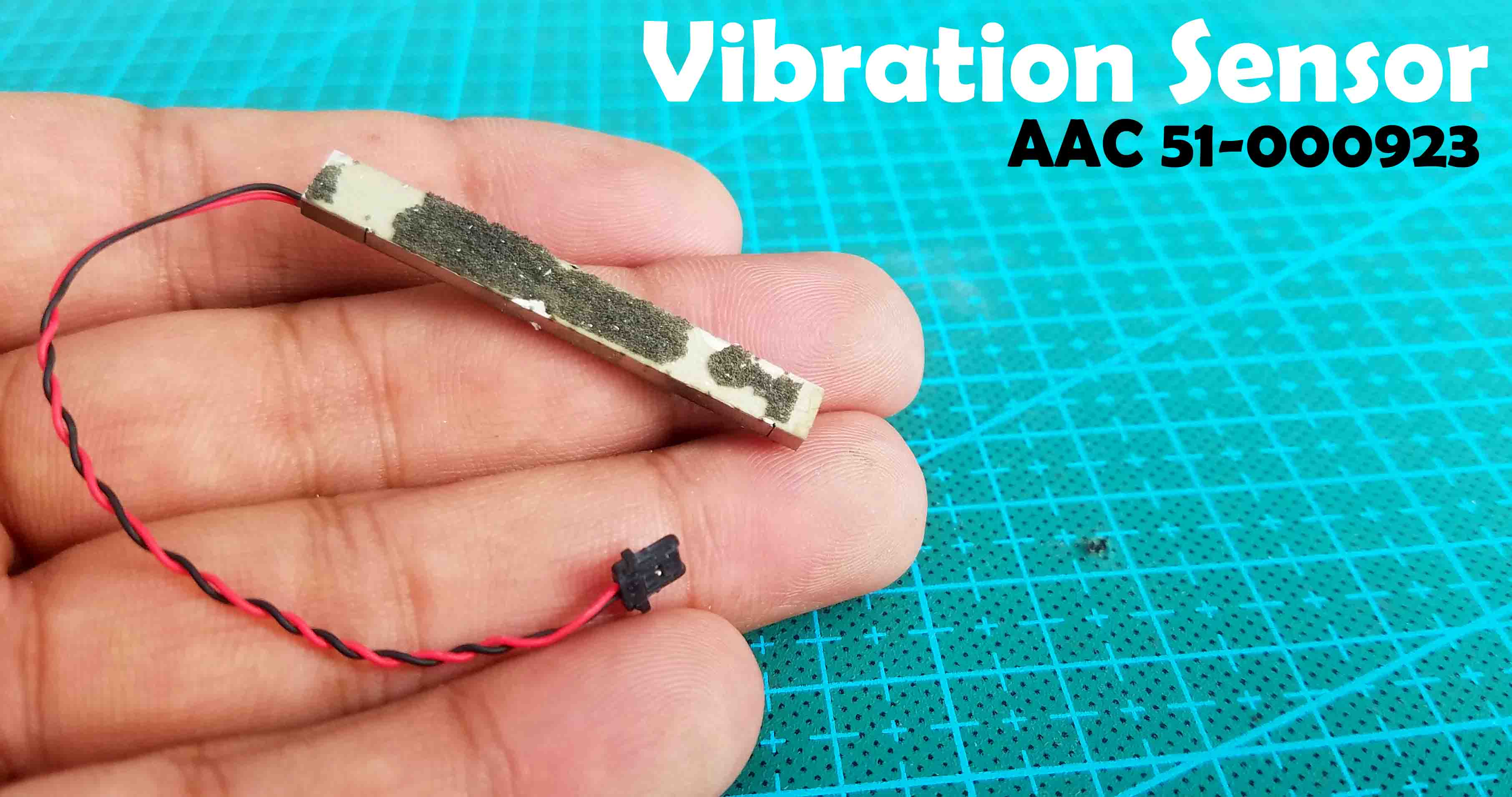This is also known as the Shock Sensor and when it vibrates, it produces a weak AC Analog voltage output which can be converted into digital using the Arduino’s Analog input pins. I will use three different programs to explain how this sensor can be used to detect vibrations. In the first two examples we will detect the vibration and display the vibration value on the Serial monitor. These two examples cover the simple analog pin reading technique and the Pulse-in technique. In the third example, we will control three different LEDs, each LED turns ON when a predefined vibration value is exceeded. Now, you have got the idea of what you are going to learn after reading this article. Without any further delay, let’s get started!!!

Arduino Uno

Arduino Nano

SW-420 Vibration Sensor

Traffic LED Module

Other Tools and Components:

Top Arduino Sensors:

Super Starter kit for Beginners

Digital Oscilloscopes

Variable Supply

Digital Multimeter

Soldering iron kits

PCB small portable drill machines

## Vibration Detector AAC 51-000923:

The AAC 51-000923 vibration sensor is made of a piezoelectric ceramic long panel. This sensor can be used in two ways; It can be subjected to severe vibration and voltage output taken from it, or an alternating voltage can be applied to it and the part can be vibrated. In other words, this piece can be both in the producer mode and in the consumer mode.

## What is a Vibration Sensor?

Vibration sensors are sensors that can measure, display, and analyze linear velocity, displacement and acceleration. Although the human senses are poor at perceiving vibration, the vibration of a device can be a warning sign for the condition of that device. Using vibration sensors, an abnormal vibration of an industrial machine can be detected in time and repaired before the machine breaks down; In other words, some failures are both costly and time consuming. Therefore, measuring vibration reduces costs by increasing productivity. Vibration analysis is used as a tool to detect and locate errors.

Vibration sensors are capable of measuring vibration based on mechanical or optical principles. Since there is no sensor to measure vibration directly, vibration is measured using mechanical or optical quantities. Vibration sensors fall into several categories; Active and inactive, relative and absolute. Also, based on the frequency range, signal dynamics and other matters related to the quality of the measured data are distinguished from each other.

applications

security systems

Smart appliances

Piezo generator voltage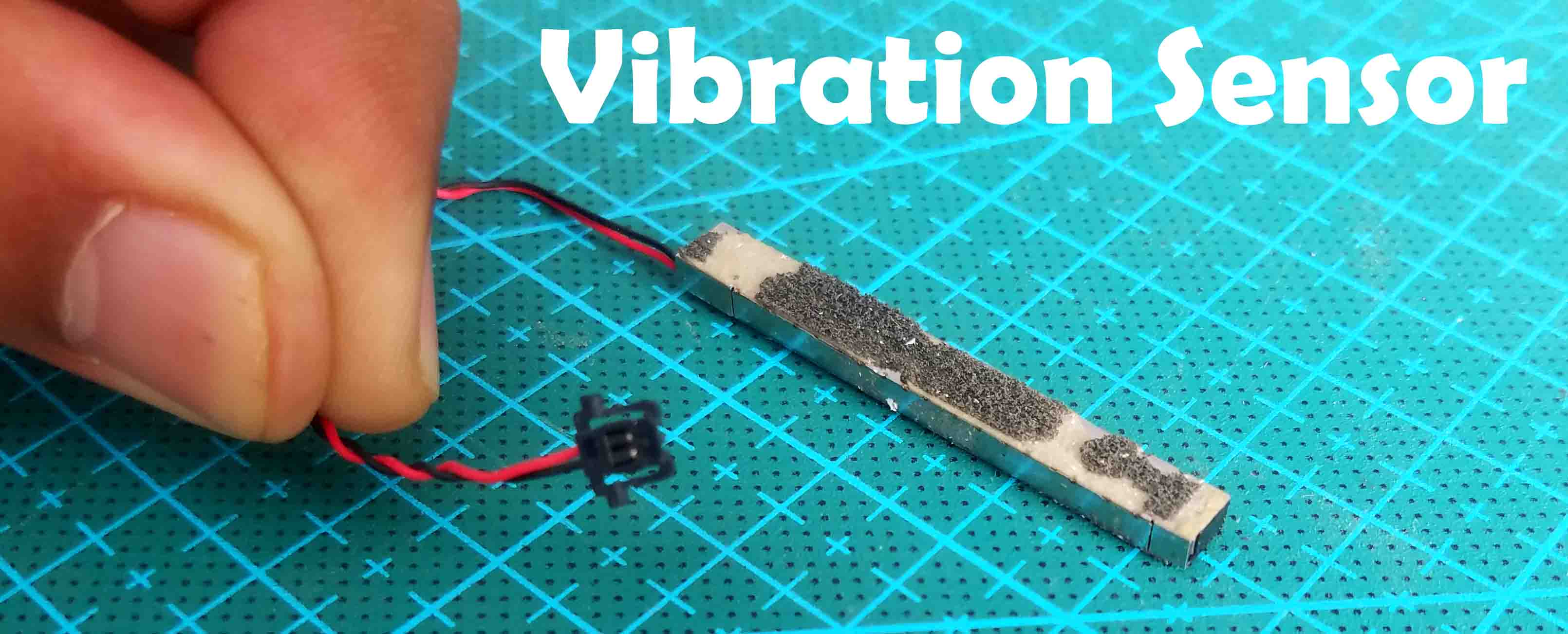This is the AAC 51-000923 Vibration Sensor. This sensor is 43mm long and has two wires Red and Black. This sensor is also provided with a connector which I am going to cut as I don’t need this and I will connect two male type jumper wires.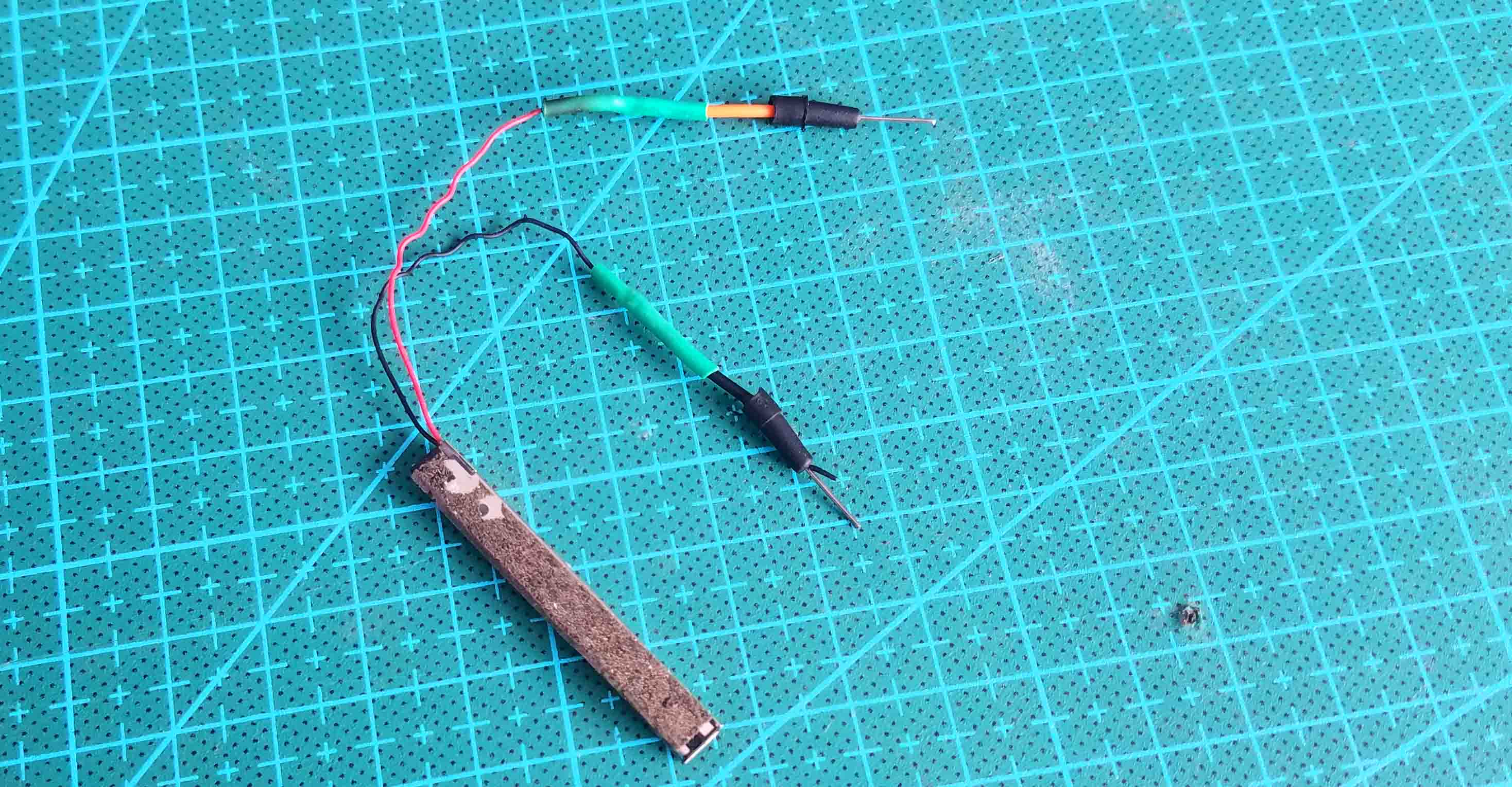As you can see my Vibration Sensor is ready and now I can easily interface this with the Arduino and other microcontroller boards using only these two wires.

## Vibration Sensor Interfacing with Arduino:Connect the Black wire of the Vibration Sensor with the Arduino’s GND and the Red wire with the Arduino’s Analog Pin A0. That’s all about the interfacing. Now, let’s take a look at the first example code.

## Vibration Sensor Arduino Code Exmaple1:

### Code Explanation:

int vib_sensor = A0;

The vibration sensor is connected with the Arduino’s Analog pin A0.

int vib_data = 0;

Next, I defined a variable of the type integer for storing the value coming from the Analog pin A0 to which the Vibration Sensor is connected.

void setup() {

// put your setup code here, to run once:

Serial.begin(9600);

Inside the void setup() function, I activated the Serial communication and selected 9600 as the baud rate.

pinMode(vib_sensor, INPUT);

Next, I set the Analog pin A0 as the input. As we are going to read the Vibration Sensor using the Analog pin A0.

}

void loop() {

// put your main code here, to run repeatedly:

Serial.println(vib_data);

delay(100);

}

Inside the void loop() function we simply read the vibration sensor and store the value in variable vib_data and then finally we print the value on the Serial monitor. So, that’s all about the exampe1 code.

### Testing Vibration Sensor:

Connect Arduino with the Laptop, select the desired com port and upload the program. When you are done with uploading the program, open the serial monitor and make sure the 9600 baud rate is selected. You can start by gently taping the vibration sensor and you will see a change in the values on the Serial Monitor, with this simple technique you can detect small vibrations.Now let’s go ahead and take a look at another example code based on the pulse-in technique.

## Vibration Sensor Arduino Code Exmaple2:

I am using the same analog pin A0. This time I am using the pulseIn() function to measure the vibration. The pulseIn() function measures the time period of a high or low pulse input signal. The if condition checks if any vibration is detected then turn on the LED. While the Arduino is still connected with the Laptop, go ahead and upload this code. When you are done with uploading the code, next open the Serial monitor and make sure the correct baud rate is selected which is 9600 in my case.With this code I wasn’t able to detect low vibration. When I tap the sensor it gives me the value and then goes back to zero. I like it. It can be used as the shock sensor, it can be ideal in situations where medium and strong hits are need to be monitored. It can also detect low vibrations but not as efficiently as the SW-420 Vibration Sensor. Now, let’s go ahead and take a look at the example3.

## Vibration Intensity Monitoring with Arduino: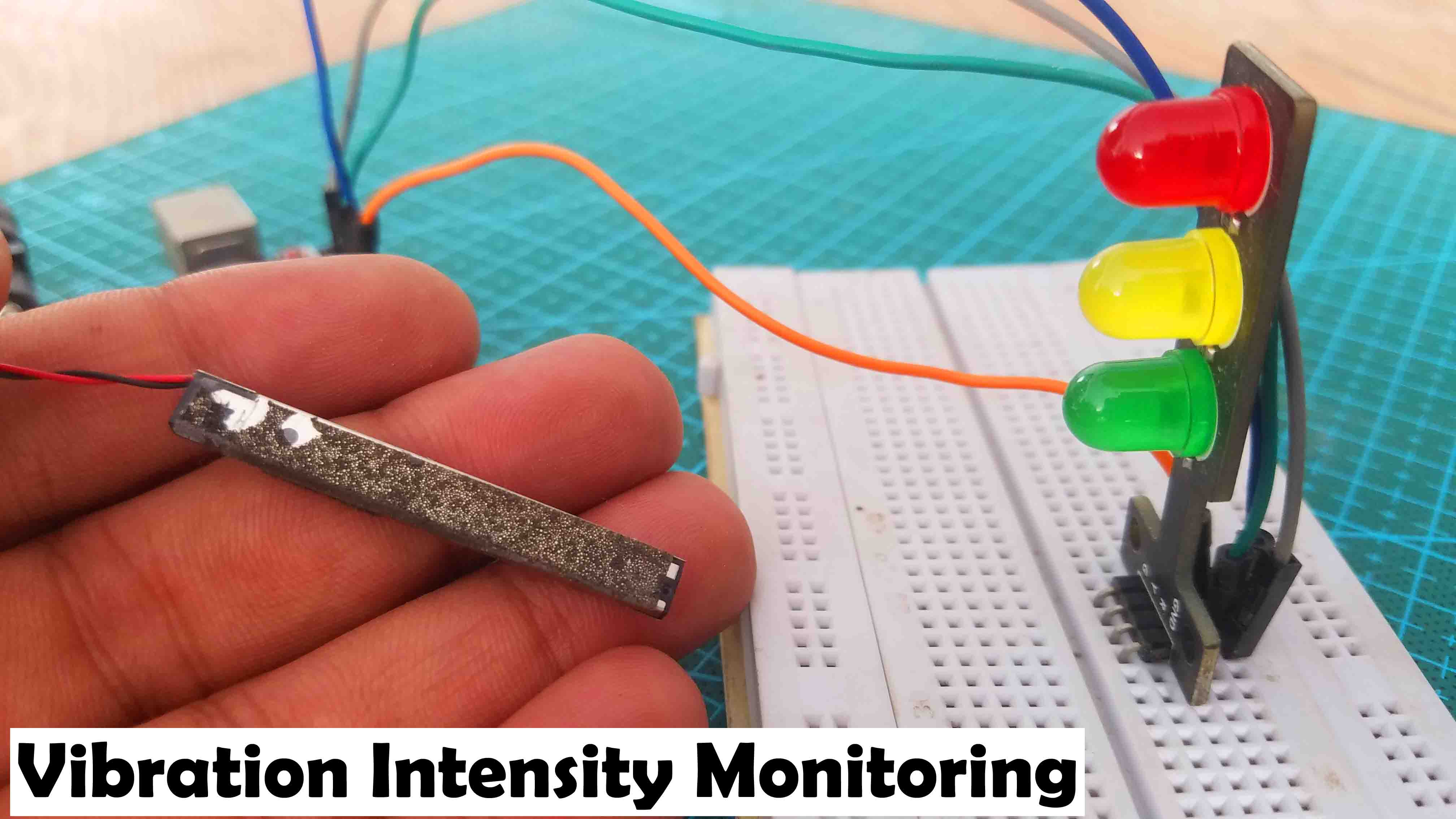This time I added this Traffic LED module with the Arduino to show the vibration intensity. The Green, Yellow, and Red LEDs represent low, medium, and high vibration. Each LED is 5 volts so; there is no need to add any current limiting resistors.

### Vibration Sensor and Led Module Interfacing with Arduino:

The connection of the Vibration Sensor with the Arduino remains exactly the same.

• The GND pin of the Traffic LED module is connected with the GND pin of the Arduino.
• The Red LED is connected with the Arduino’s pin number 13,
• The Yellow LED is connected with the Arduino’s pin number 12, and
• The Green LED is connected with the Arduino’s pin number 11.

Our hardware is ready and now let’s take a look at the example code number3.

### Vibration Intensity Monitoring Arduino Code, Example3:

This code is the modified version of the code I used in example2. You can see maximum of the instructions are exactly the same. This time I defined some pins for the LEDs and in the void loop() function I added three if conditions to control these three LEDs using different vibration values. I uploaded this program and I was able to control these LEDs.